# 基于PINN的极少监督数据二维非定常圆柱绕流模拟

04/12 11:44

• 会议论文代码下载地址

https://github.com/tianshao1992/PINNs_Cylinder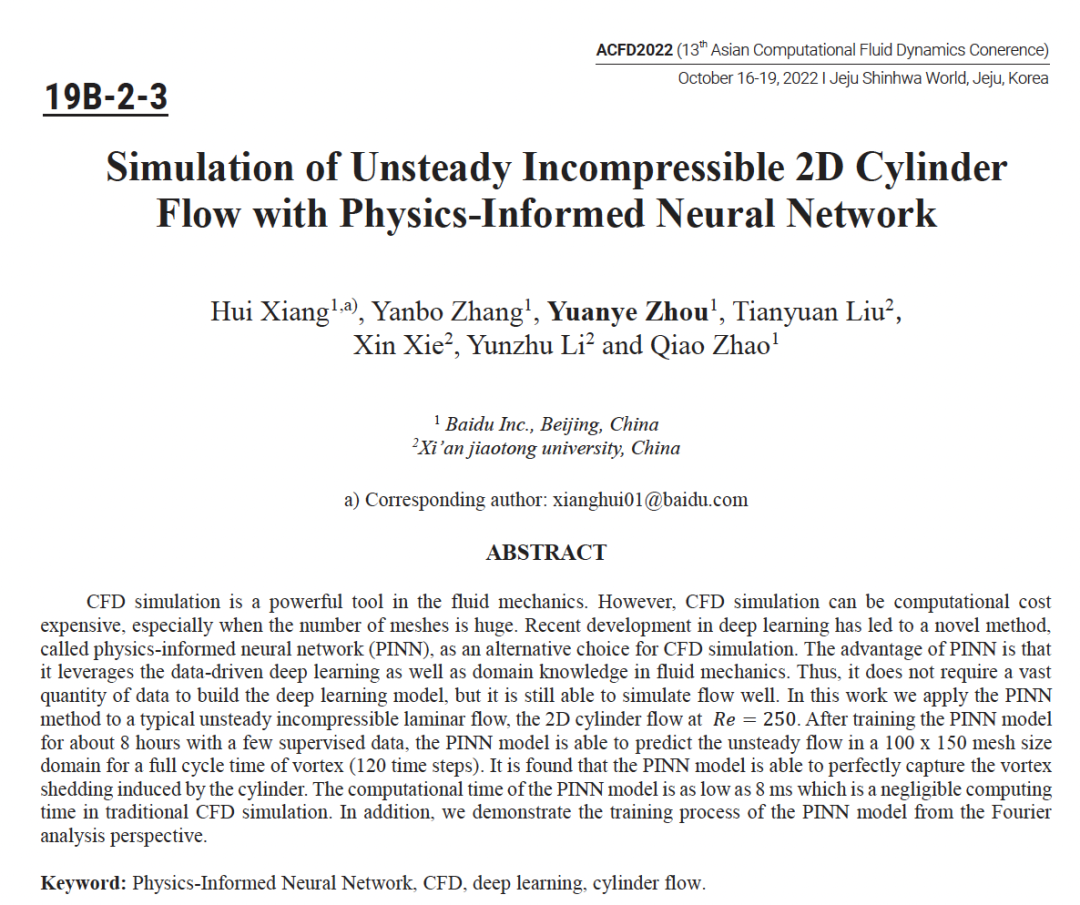# 研究方法

## 二维非定常不可压圆柱绕流问题描述

$\mathrm{\nabla}·u=0$ (1)

$\frac{\partial\mathrm{u}}{\partial t}+\mathrm{u}∙∇\bullet+\frac{1}{\rho}\mathrm{\nabla}\mathrm{p}-ν\mathrm{\nabla}2u-f = 0$ (2)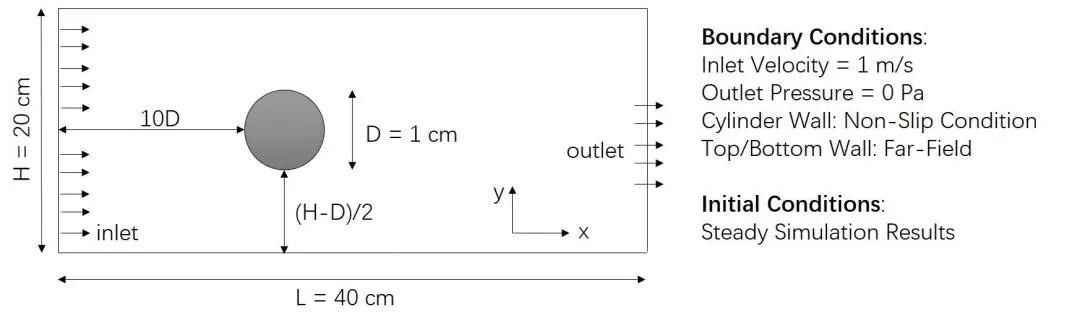# 物理信息约束神经元网络（PINN）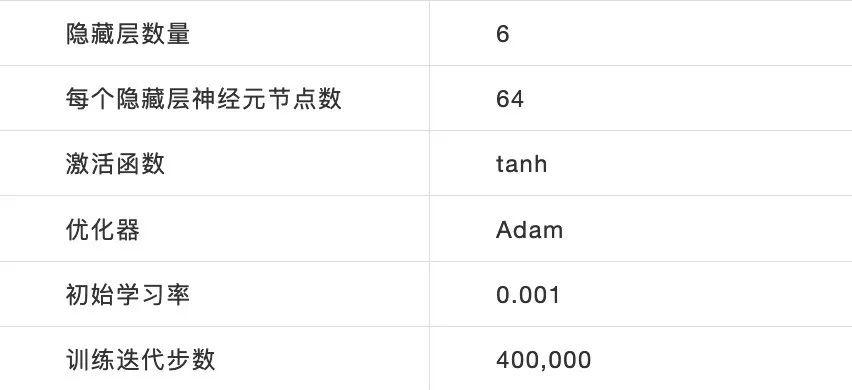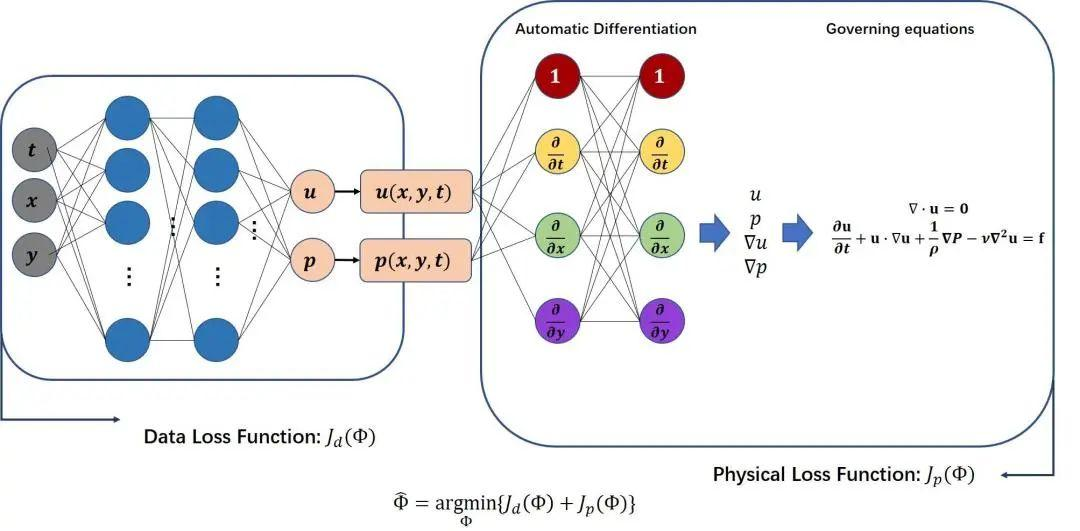## PINN的损失函数

$J=J_d+\omega J_p$(3)

$J_d=\frac{1}{N_d}\sum_{i=1}^{N_d}\left|Y_{pred}^i-Y_{true}^i\right|^2$(4)

$J_p=J_{PDE}+J_{BC}+J_{IC}$(5)

$J_{PDE}$，$J_{BC}$和$J_{IC}$的定义如下:

$J_{PDE}=\frac{1}{N_f}\sum_{i=1}^{N_f}\left|G\left({u}^i,p^i\right)\right|^2$ (6)

$J_{BC}=\frac{1}{N_{BC}}\sum_{i=1}^{N_{BC}}\left|Y_{pred}^i-Y_{true}^i\right|^2$ (7)

$J_{IC}=\frac{1}{N_{IC}}\sum_{i=1}^{N_{IC}}\left|Y_{pred}^i-Y_{true}^i\right|^2$ (8)

$J=\omega_1J_d+\omega_2J_{PDE}+\omega_3J_{BC}+\omega_4J_{IC}$ (9)

## PINN的监督点分布

$N_d=68$的监督点分布存在两种方式，a方案在圆柱前缘也布置一些点，b方案则所有点均在圆柱后方。 $N_d=34$的监督点分布存在两种方式，a方案集中在圆柱近后方，b方案则分散在圆柱近和远后方。$N_d=16$和$N_d=4$的监督点分布的区别在于$N_d=4$仅在圆柱表面均分分布4个监督点。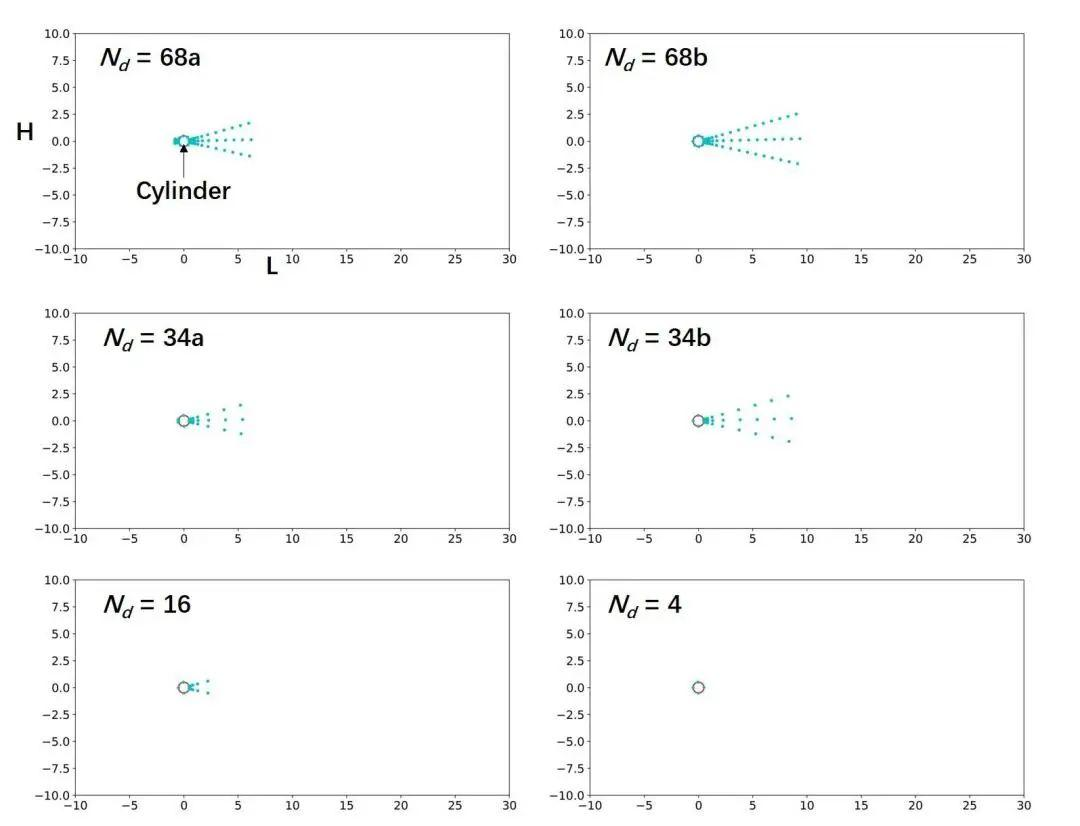# 研究结果

## PINN的训练过程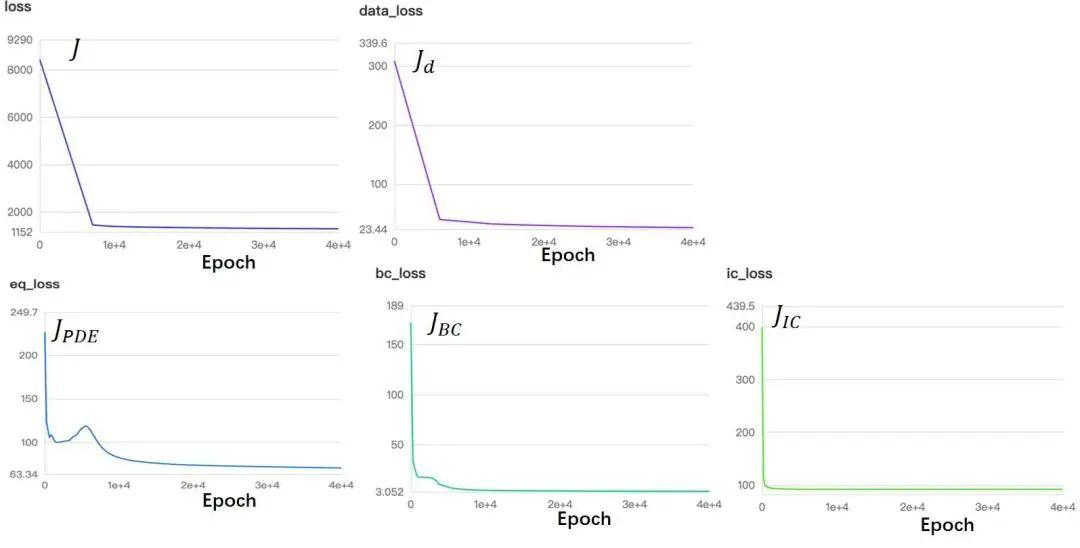## PINN与FVM对比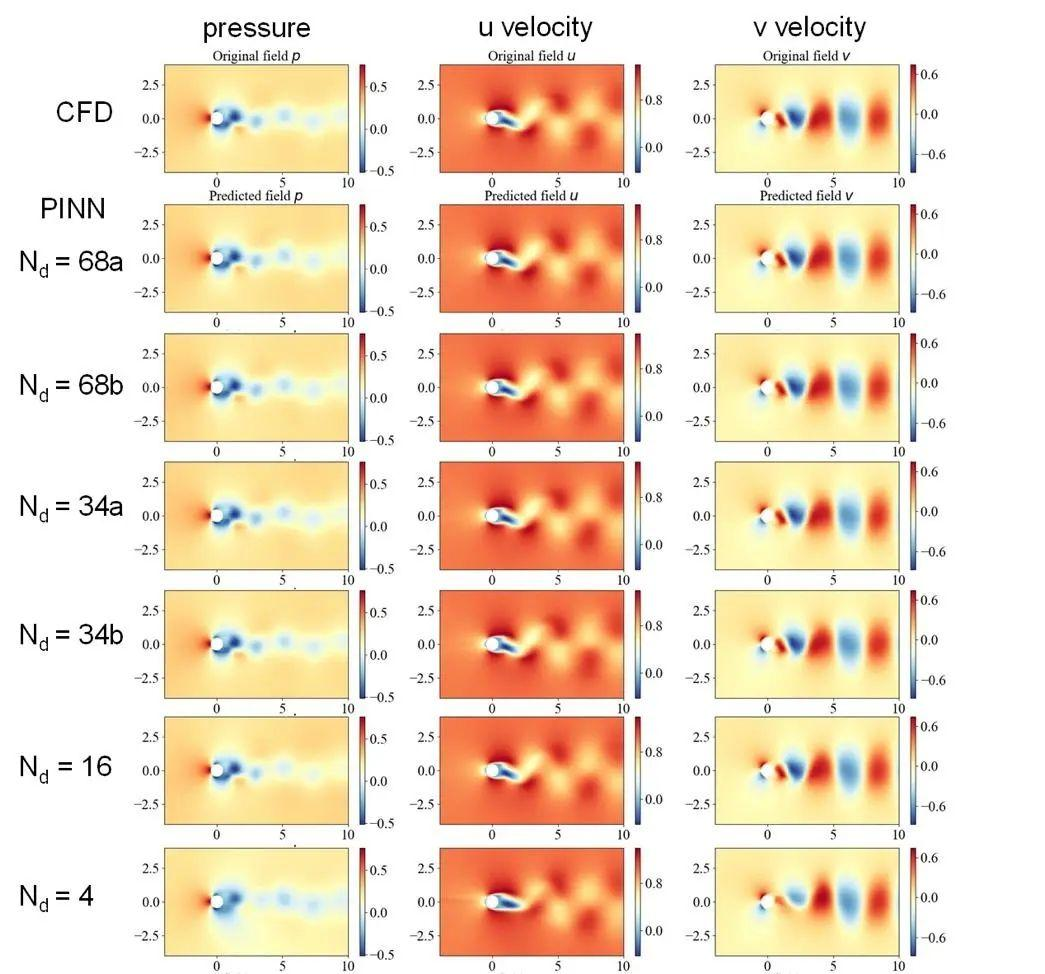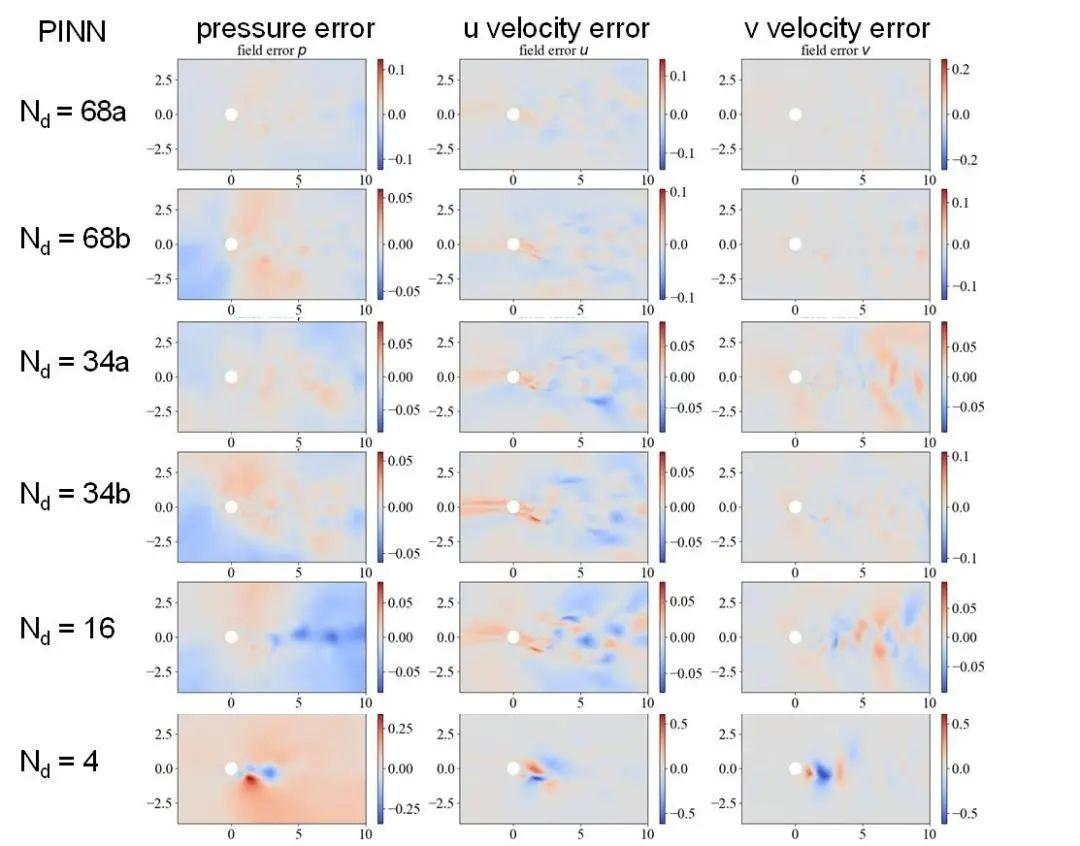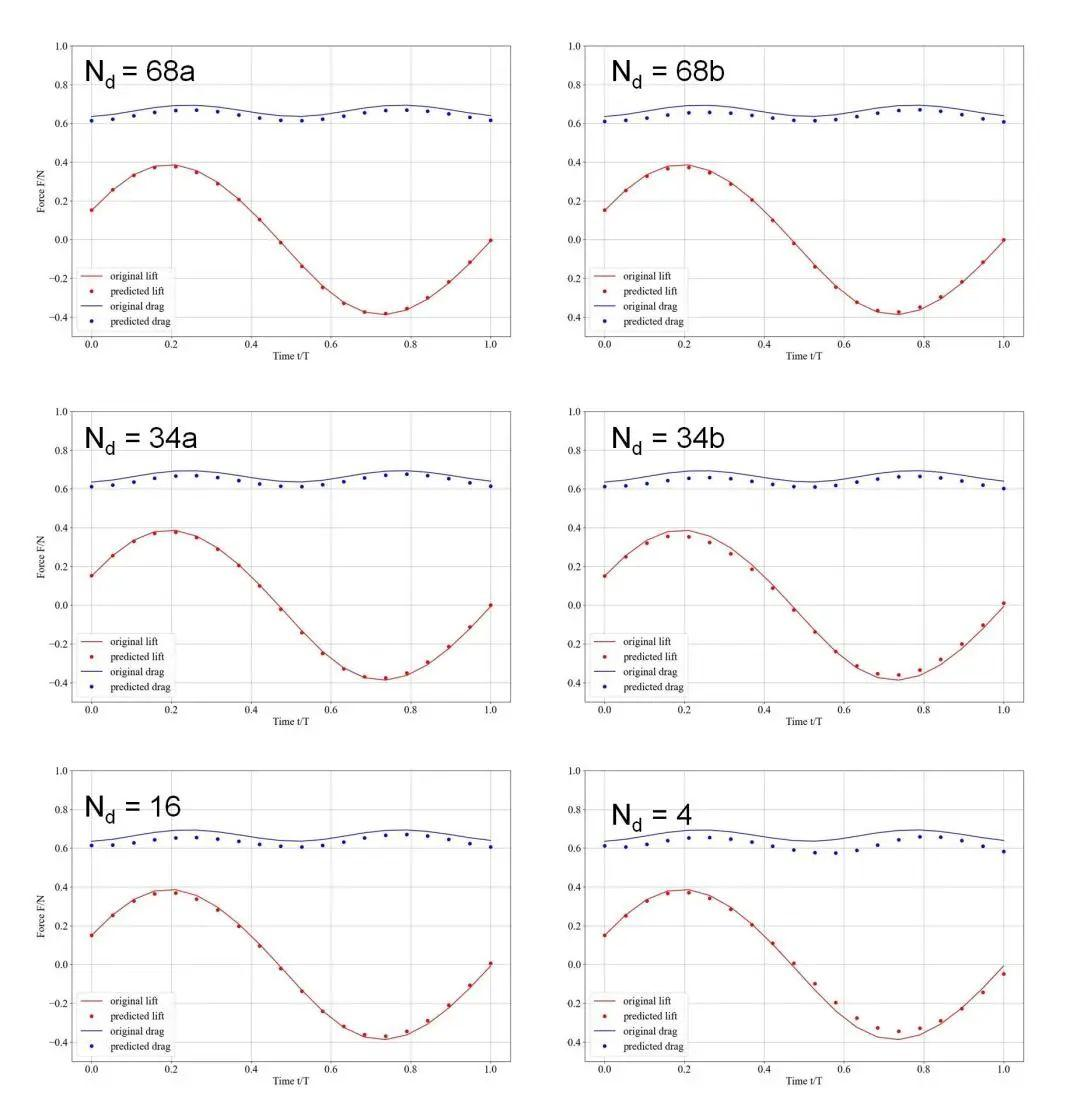# 结果讨论

 Teigland, R., & Eliassen, I. K. (2001). A multiblock/multilevel mesh refinement procedure for CFD computations. International journal for numerical methods in fluids, 36(5), 519-538.
 Volk, A., Ghia, U., & Stoltz, C. (2017). Effect of grid type and refinement method on CFD-DEM solution trend with grid size. Powder Technology, 311, 137-146.
 Raissi, M., Perdikaris, P., & Karniadakis, G. E. (2017). Physics informed deep learning (part i): Data-driven solutions of nonlinear partial differential equations. arXiv preprint arXiv:1711.10561.
 https://aistudio.baidu.com/aistudio/projectdetail/4178470

2. 飞桨AI for Science流体力学公开课第一期：
https://aistudio.baidu.com/aistudio/course/introduce/27926

1. 飞桨AI for Science共创计划：

2. 飞桨PPISG-Science小组：